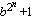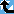# Generalized Fermat Divisors (base=10)

The Prime Pages keeps a list of the 5000 largest known primes, plus a few each of certain selected archivable forms and classes. These forms are defined in this collection's home page.

The numbers Fb,n =(with b an integer greater than one) are called the generalized Fermat numbers. (In the Prime database they are denoted GF(n,b) to avoid the use of subscripts.) It is reasonable to conjecture that for each base b, there are only finitely many such primes.

As in the case of the Fermat numbers, many have interested in the form and distribution of the divisors of these numbers. When b is even, each of their divisors must have the form

k.2m+1
with k odd and m>n. For this reason, when we find a large prime of the form k.2n+1 (with k small), we usually check to see if it divides a Fermat number. For example, Gallot's Win95 program Proth.exe has this test built in for a few choices of b.

The number k.2n+1 (k odd) will divide some generalized Fermat number for roughly 1/k of the bases b.

###Record Primes of this Type

rankprime digitswhowhencomment
111 · 215502315 + 1 4666663 L4965 Jan 2023 Divides GF(15502313, 10) [GG]
237 · 210599476 + 1 3190762 L4965 Jun 2022 Divides GF(10599475, 10)
317 · 28636199 + 1 2599757 L5161 Feb 2021 Divides GF(8636198, 10)
4135 · 24162529 + 1 1253049 L5178 Jul 2023 Divides GF(4162525, 10)
59 · 23497442 + 1 1052836 L1780 Oct 2012 Generalized Fermat, divides GF(3497441, 10)
61005 · 23420846 + 1 1029781 L2714 Aug 2017 Divides GF(3420844, 10)
7357 · 23273543 + 1 985438 L5237 Jul 2022 Divides GF(3273542, 10)
8169 · 22545526 + 1 766282 L2125 Jan 2015 Divides GF(2545525, 10), generalized Fermat
97 · 22167800 + 1 652574 g279 Apr 2007 Divides Fermat F(2167797), GF(2167799, 5), GF(2167799, 10)
103 · 22145353 + 1 645817 g245 Feb 2003 Divides Fermat F(2145351), GF(2145351, 3), GF(2145352, 5), GF(2145348, 6), GF(2145352, 10), GF(2145351, 12)
1125 · 22141884 + 1 644773 L1741 Sep 2011 Divides Fermat F(2141872), GF(2141871, 5), GF(2141872, 10); generalized Fermat
1245 · 22014557 + 1 606444 L1349 Feb 2012 Divides GF(2014552, 10)
13657 · 21998854 + 1 601718 L2520 Oct 2013 Divides GF(1998852, 10)
14175 · 21962288 + 1 590710 L2137 Jul 2013 Divides GF(1962284, 10)
15135 · 21515894 + 1 456332 L1129 Jan 2013 Divides GF(1515890, 10)
1617 · 21388355 + 1 417938 g267 Sep 2005 Divides GF(1388354, 10)
17107 · 21337019 + 1 402485 L2659 Jan 2012 Divides GF(1337018, 10)
181389 · 21335434 + 1 402009 L1209 Jan 2015 Divides GF(1335433, 10)
1915 · 21276177 + 1 384169 g279 Feb 2006 Divides GF(1276174, 3), GF(1276174, 10)
2029 · 21152765 + 1 347019 g300 Sep 2005 Divides GF(1152760, 10)

###References

BR98
A. Björn and H. Riesel, "Factors of generalized Fermat numbers," Math. Comp., 67 (1998) 441--446.  MR 98e:11008 (Abstract available)
DK95
H. Dubner and W. Keller, "Factors of generalized Fermat numbers," Math. Comp., 64 (1995) 397--405.  MR 95c:11010
RB94
H. Riesel and A. Börn, Generalized Fermat numbers.  In "Mathematics of Computation 1943-1993: A Half-Century of Computational Mathematics," W. Gautschi editor, Proc. Symp. Appl. Math. Vol, 48, Amer. Math. Soc., Providence, RI, 1994.  pp. 583-587, MR 95j:11006
Riesel69
H. Riesel, "Some factors of the numbers Gn = 62n + 1 and Hn = 102n + 1," Math. Comp., 23:106 (1969) 413--415.  MR 39:6813